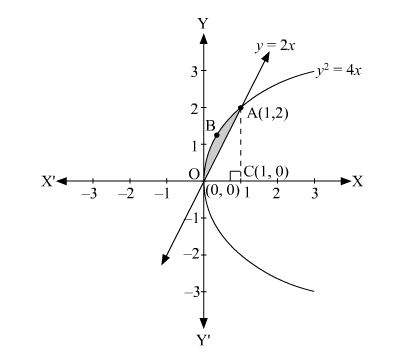# Area lying between the curve

Question:

Area lying between the curve $y^{2}=4 x$ and $y=2 x$ is

A. $\frac{2}{3}$

B. $\frac{1}{3}$

C. $\frac{1}{4}$

D. $\frac{3}{4}$

Solution:

The area lying between the curve, $y^{2}=4 x$ and $y=2 x$, is represented by the shaded area $\mathrm{OBAO}$ asThe points of intersection of these curves are O (0, 0) and A (1, 2).

We draw AC perpendicular to x-axis such that the coordinates of C are (1, 0).

∴ Area OBAO = Area (OCABO) – Area (ΔOCA)

$=\int_{0}^{1} 2 \sqrt{x} d x-\int_{0}^{1} 2 x d x$

$=2\left[\frac{x^{\frac{3}{2}}}{\frac{3}{2}}\right]_{0}^{1}-2\left[\frac{x^{2}}{2}\right]_{0}^{1}$

$=\left|\frac{4}{3}-1\right|$

$=\frac{1}{3}$ square units

Thus, the correct answer is B.